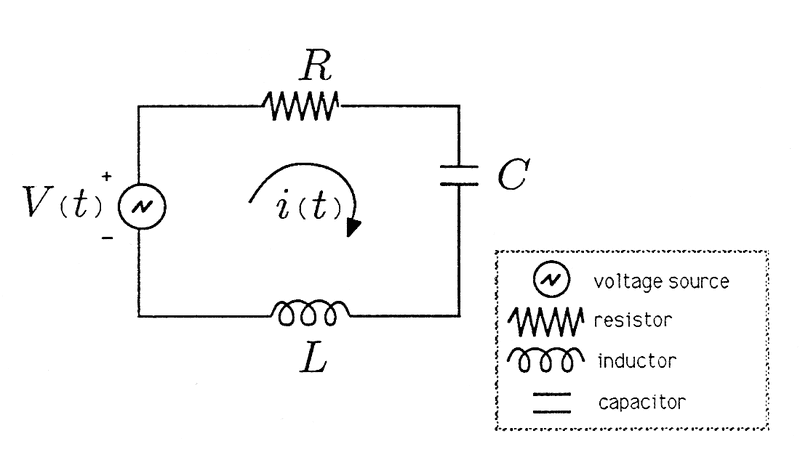# RLC AC Circuit Question

Voltage V(t)=V1 Re{eiwt} produces a current I(t)=I1 Re{e(iwt+θ). Determine I1 and θ.

The circuit is AC so do I treat it the same as an SHM question? Is π/2? And what do I need to do to find I1? I don't know where to start.

ehild
Homework Helper
What is the circuit? You need its complex impedance to find I1 and θ.

ehild

Ohh. The circuit is like this one:with no values given.

ehild
Homework Helper
What is the impedance in terms of R, L, and C?

ehild

z = R + iωL +1/iωC ?

What is the impedance in terms of R, L, and C?

ehild

Last edited:
ehild
Homework Helper
OK, collecting the imaginary terms, it is Z=R+i(ωL -1/ωC ). This is a complex number. You can write it in exponential form:

Z=|Z|e (How?).

The complex voltage is V=V1eiωt

Use the equation I=V/Z to find the complex current.

ehild

Last edited:
OK, collecting the imaginary terms, it is Z=R+i(ωL -1/ωC ). This is a complex number. You can write it in exponential form:

Z=|Z|eiωt (How?).

The complex voltage is V=V1eiωt

Use the equation I=V/Z to find the complex current.

ehild
I=V0/|Z| ?

But that gives me I0= V0/|Z|ei(ωt+θ).

Last edited:
ehild
Homework Helper
I=V0/|Z| ?

But that gives me I0= V0/|Z|ei(ωt+θ).

You used I1 and V1 for the amplitudes in the original post.

I=V/Z . Z=|Z|e

That means

I0ei(ωt+θ)=V0ei(ωt)/[|Z|e]

Simplify with ei(ωt).

I0e=V0/[|Z|e]=[V0/|Z|]e-iψ

The magnitudes and phases have to be the same on both sides.

ehild